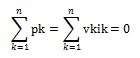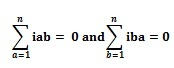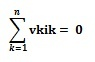# What is Tellegen’s Theorem : Working & Its Applications

In network analysis, knowing about the network theorem is very important for circuit analysis. So there are different network theorems available like Superposition, Tellegen’s, Norton’s, maximum power transfer, Thevenin’s, Compensation, Substitution, Reciprocity, Millman’s & Miller’s theorem. But understanding each network theorem is very important because each theorem is used for specific applications. This article discusses one of the types of network theorems like Tellegen’s theorem, working with examples.

## What is Tellegen’s Theorem?

Tellegen’s theorem is a type of network theorem which was invented & published by a Dutch electrical engineer namely Bernard D.H. Tellegen in the year 1952. In network analysis, this theorem is a very essential theorem as compared to other theorems because most of the network theorems can be derived by using this theorem.

This network theorem mainly depends on Kirchhoff’s law. So, this theorem can be simply applied to the network which follows Kirchhoff’s law. This network theorem is used in circuits that have linear, nonlinear, active, passive, time-variant, or non-variant elements.

Tellegen’s Theorem Statement is, “the summation of direct power used by different elements within different branches is equivalent to zero (0) for any type of network”. This theorem works on the principle of the conservation energy principle, so used in biological and chemical applications to discover the active performance of the physical network and also used to design filters in signal processing,.

#### Steps to Solve Telegen’s Theorem

The following steps need to follow to solve any electrical network using Tellegen’s theorem.

• First, we need to locate the branch voltage drops in an electrical network.
• Find the equivalent branch currents through conventional analysis techniques
• Then, this theorem can be justified by summing up the products of voltages & currents in all branches.

### Tellegen’s Theorem for AC Circuits

The Tellegen’s theorem basic concept is being the same in a.c. or d.c circuits. For the a.c circuit’s application, it can be stated as, in any active or passive, linear or non-linear, time-variant network, exited through alternating sources, the summation of instant or complex power of the source is zero.

For analysis of this theorem, consider the network with ‘n’ number of elements. The instant current flows throughout the element is i1, i2, i3,i4 …., in whereas the instant voltage in this branch is v1, v2, v3,v4 …., vn.

So, the instant current & voltage for element-1 are i1 & v1 and instant power (p1) utilized through this element is ‘v1i1’.

p1 = v1* i1

For element2, the instant power (p2) is

p2 = v2* i2

Likewise, the instant power of nth element is (pn) is

pn = vn* in

Based on the Tellegen’s theorem, all instant power summation is ‘0’. So, we need to sum all instant power like p1, p2, p3,p4 …., on.

p1 + p2 + p3 +p4 … + pn = 0

v1 i1+ v2 i2 + v3 i3 + v4i4… + vn in = 0

The above equation can be written in general form for the kth branch as;In the above equation,

‘n’ is the total number of branches within the network

‘vk’ is an instant voltage of the ‘kth’ branch

‘ik’ is an instant current of ‘kth’ branch

‘pk’ is an instant power of the ‘kth’ branch

If we consider the below figure as an AB branch that is equivalent to a k branch.

Thus, instant voltage vk = va – vb

The instant flow of current throughout a to b branch is ‘ik’;

ik = iab

As a result, the instant power like pk is;

pk = vk* ik = (va – vb) iab …..(I)

Now, we need to consider the instant current in opposite direction from b to a;

iab = – iab

Instant voltage;

vk = vb – va

The instant power ‘pk’ is;

pk = vk ik = (vb – va) iba ……(ii)

By summing above i & ii equations, we can get

2vk ik = (va – vb) iab + (vb – va) iba

vk ik = 1/2 [(va – vb) iab + (vb – va) iba] ……..(iii)

According to Kirchhoff’s current law, the algebraic summation of current is zero at a node of the circuit.

Hence,If we substitute this value in the above equation (iv), we getSo, it is verified that the summation of delivered power to the network is ‘0’. So, Tellegen’s theorem is proved. It is also explained that the sum of absorbed power through the network elements is equivalent to the sum of delivered power through the sources.

### Tellegen’s Theorem Solved Problems

The example problems of Tellegen’s theorem are solved below.

Example1: Verify Telegen’s theorem for the following DC network.

Apply KCL at node

V-20/2+V/2+V-10/2 = 0

(V-20+V+V-10)/2 =0

3V = 30

V = 30/3 = 10Volts

When current flowing through the circuit then

I1 = 20-V/2 = 20-10/2 =10/2 = 5A

I2 = V/2 = 10/2 = 5A

I3 = V-10/2 = 10-10/2 = 0

Power supplied by 20V source is,

P = V x I => 20×5 = 100 Watts

Power absorbed by passive elements in the network is

P = I^2 R => 5^2×2+ 5^2×2

25 x 2+25 x 2 = 100Watts

Therefore, power supplied = power absorbed

So, the algebraic sum of all powers in a network is equal to zero.

Example2:

Using Tellegen’s theorem, find the voltage across 6A current source for the following circuit.

First, we need to calculate the current or voltage flowing throughout the circuit by applying KVL or KCL to the above network.

By applying KVL to the loop-2, we can get

-12 = 8I2 – 6I1

The flow of current throughout the branch including current source are I1 =6A;

Substitute this ‘I1’ value in the above equation; we can get ‘I2’ value.

-12 = 8I2 – 6I1

We know that I1 = 6A

-12 = 8I2 – 6(6)

-12 = 8I2 – 36

8I2 = 36 – 12 => 24

I2 = 3A

Flow of current throughout the branch-2 is;

I12 = I1 – I2 => 6 – 3 = 3A

I12 = 3A

Now, w need to find the power for every branch

P1 = V I1 = V x 6 = 6 x V

P2 = R1 I12^2 = 6 x 9 = 54W

P3 = R2 I2^2 = 2 x 9 = 18W

P4 = V I2 = -12 x 3 = -36W

Here, energy sources are available in two branches. Thus, these branches can be considered as power delivering branches. Set the positive (+ve) sign to the instant power.

The remaining branches have only resistors. Thus, these are power absorbing branches & set the negative (-) sign to the instant power.

Both the powers like P1 & P4 have a positive sign whereas powers P2 & P3 have a negative sign.
Now, we need to add all instant power.

P1 – P2 – P3 + P4 = 0W

P1 – 54 – 18 + 36 = 108W

The power transmitted throughout the 6A current source is 108W. Thus the voltage across the 6A source of current can be calculated through the following equation.

P1 = V I

108W = V x 6A

V = 108/6 = 18V

So, the voltage across the 6A current source is 18V.

How are KCL KVL and Tellegen’s theorem related?

The consequence of Kirchhoff’s laws like KVL & KCL is Tellegen’s theorem. This theorem is verified through KVL or KCL.

Can we verify Thevenin’s theorem both on AC and DC circuits?

Yes, we can verify Thevenin’s theorem both on AC & DC circuits. Similar to the DC circuits, this theorem can be applied to the AC circuits which contain linear elements such as resistors, capacitors & inductors. Similar to equivalent Thevenin’s resistance, equivalent impedance can be obtained by changing all voltage sources through their inside impedances.

Which theorem is applicable for the linear and nonlinear circuits?

Substitution theorem is applicable for linear and nonlinear circuit

How do you use Tellegen’s theorem?

Tellegen’s theorem can be used to design the filters & complex operating systems.

### Applications

The applications of Tellegen’s Theorem include the following.

• Tellegen theorem is used to examine difficult network systems like electrical circuits, metabolic & biological networks, chemical processes & pipeline transport networks.
• Tellegen’s theorem is used to design filters in the digital signal processing (DSP) system.
• Used in biological & chemical processes.
• Used in reaction network structure analysis & topology.
• Used in different oil and chemical plants to determine the complex system’s stability.
• This theorem is applicable for the circuits which have linear/nonlinear, time-variant/non-variant, & active/passive elements.

Thus, this is all about an overview of Tellegen’s Theorem, derivation, example problems, and its applications. The limitations of Tellegen’s theorem are, for Tellegen’s theorem verification, KVL & KCL equations are used. This theorem mainly works on the law of energy conservation principle. This theorem is used in a number of network systems. Here is a question for you. What is the superposition theorem?